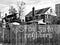# Perceptron from (Almost) Zero and 3D Visualization

In a previous post i’ve introduced the idea the Perceptron algorithm to solve an specific problem (the AND gate). The ideia was to get a feel of how the algorithm works, and now we can generalize it to solve any linearly separable problem (that’s why the ‘almost’ in the title).

The main adjust to our previous work on Perceptron to solve AND gate is to generalize the number of inputs that our algorithm will receive, so it can handle datasets with an arbitrary number of inputs. …

# K-NN from scratchPhoto by Bill Nino on Unsplash

K- Nearest Neighbors is one of the most used algorithms in classification problems. implementing it from scratch is a great programming exercise and can give us a lot of insights about data processing, learning optimization, and general statistical learning insights, that’s what I’ll try to accomplish in this article and it hopefully will lead us to a second part to discuss PCA analysis.

# How it works

If you are familiar with libraries such as scikit-learn, you know how easy can be to implement a machine learning algorithm. But there’s a lot happening behind a classification or regression algorithm, and understanding the way those…

# Implementing the Perceptron Learning Algorithm to Solve AND Gate in Python

If you searched for Neural Networks, Deep Learning, Machine Learning, or anything that has to do with Artificial Intelligence, you’ve probably heard about the Perceptron. The1958’s famous algorithm created by Frank Rosenblatt is the landing mark in Neural Networks and consequently Deep Learning, and understanding the way it works (i.e., the math behind it) is essential to go a step further to more complex IA models, specifically in Neural Networks.

# AND gate as a classification problem

We can say that all a perceptron does is apply some transformations (mathematical functions) in a set of inputs to represent them in a significant way in space (actually, that’s…## Neto Figueira

Physicist, Data Scientist